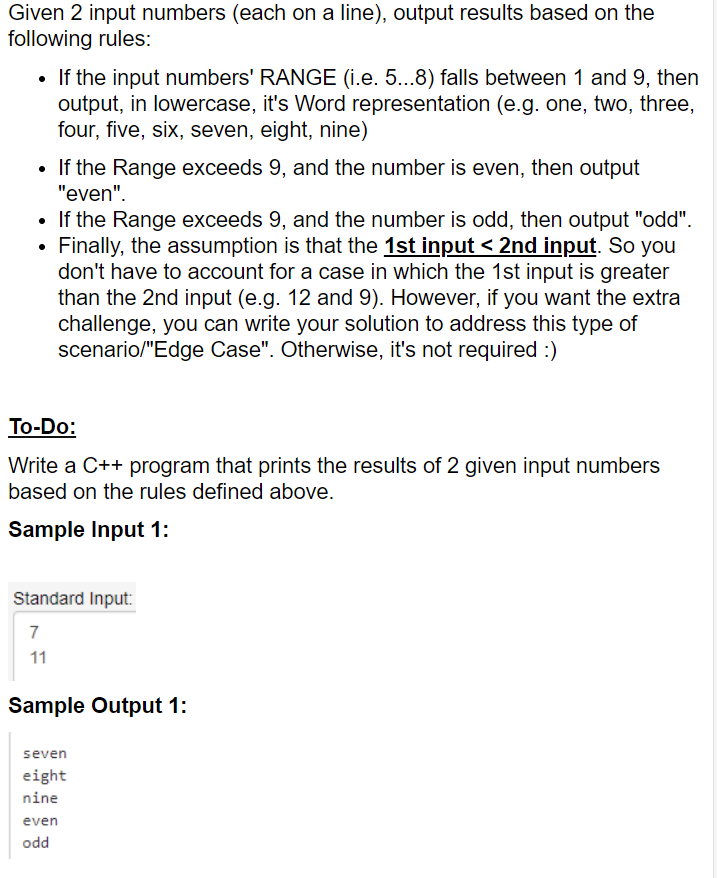# Question please use c++, thank you! Given 2 input numbers (each on a line), output results based on the following rules: • If the input numbers' RANGE (i.e. 5...8) falls between 1 and 9, then output, in lowercase, it's Word representation (e.g. one, two, three, four, five, six, seven, eight, nine) • If the Range exceeds 9, and the number is even, then output "even". • If the Range exceeds 9, and the number is odd, then output "odd". Finally, the assumption is that the 1st input <2nd input. So you don't have to account for a case in which the 1st input is greater than the 2nd input (e.g. 12 and 9). However, if you want the extra challenge, you can write your solution to address this type of scenario/"Edge Case". Otherwise, it's not required :) To-Do: Write a C++ program that prints the results of 2 given input numbers based on the rules defined above. Sample Input 1: Standard Input 7 11 Sample Output 1: seven eight nine even oddSMOO5D The Asker · Computer Science